# Abstract

In this paper we discuss the semiclassical approximation for the thermodynamics of scalar fields. We construct a semiclassical propagator in terms of two solutions of an ordinary differential equation. The main result is an analytic (non-perturbative) expression for the partition function written in terms of known quantities.

Semiclassical approximation; Thermodynamics of scalar fields; Partition function

Semiclassical approximation for the partition function in QFT

A. BessaI; C.A.A. de CarvalhoI; E.S. FragaI; F. GelisII

IInstituto de Física, Universidade Federal do Rio de Janeiro C.P.68528, Rio de Janeiro, 21941-972, Brazil

IIService de Physique Théorique CEA/DSM/Saclay, Bât. 774 91191, Gif-sur-Yvette Cedex, France

ABSTRACT

In this paper we discuss the semiclassical approximation for the thermodynamics of scalar fields. We construct a semiclassical propagator in terms of two solutions of an ordinary differential equation. The main result is an analytic (non-perturbative) expression for the partition function written in terms of known quantities.

Keywords: Semiclassical approximation; Thermodynamics of scalar fields; Partition function

I. INTRODUCTION

Semiclassical methods are widely used in many areas of physics. In one-dimensional quantum mechanics, strong results on ordinary differential equations lead to a simple expression for the semiclassical propagator in terms of the classical solution. Using the semiclassical propagator, one can construct the whole semiclassical series, either in quantum mechanics or in quantum statistical mechanics.

Simple methods can also be applied in higher-dimensional quantum mechanical systems with radial or transverse symmetry. Even in quantum field theories, where one has to deal with partial differential equations, there are relevant examples[4-6] where the semiclassical propagator can be obtained using elementary methods.

In this paper, we discuss a semiclassical approximation for the thermodynamics of scalar fields. The method is essentially non-perturbative. Usual perturbative calculations in quantum field theories at finite temperature are plagued with infrared divergencies at high (and physical relevant) values of the coupling constant[9,10]. Involved methods have been used to circumvent that problem and to bring the validity of perturbation results closer to experimental and lattice data[11,12]. In QCD, resummation techniques work down to around 2Tc, where Tc is the deconfinement phase transition temperature.

Here, we obtain an analytic expression for the partition function in terms of two solutions of an ordinary differential equation determined by a time-dependent classical field. The paper is organized as follows: in Section II we present the usual expansion around a classical solution. Difficulties in implementing that expansion lead us to propose an expansion in fluctuations of the boundary conditions of the classical equations of motion. The whole procedure is presented in Section III. Finally, Section IV is reserved to conclusions and final remarks.

II. FLUCTUATIONS AROUND A CLASSICAL SOLUTION

The starting point is the expression of Z in terms of path integrals :where SE[f] is the euclidean action of the field:Assume for the time being that we know the solution fc(t,x) of the classical equation of motion :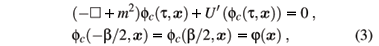where=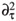+ Ñ2. The functional integration over f(t, x) in eq. (1) is dominated by field configurations in the vicinity of that classical solution. We introduce fluctuations: f(t, x) º fc(t, x)+h(t, x), and then, expand the euclidean action to second order in h(t, x) :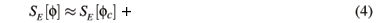The gaussian functional integration over h can be performed and the result is formally given by a determinant: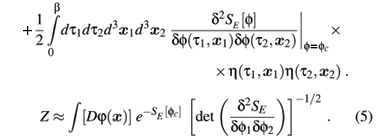The integrand is an implicit functional of j(x) through the dependence of the classical solution fc on the boundary conditions in eq. (3). In order to complete the semiclassical calculation of Z, one has to integrate over j(x). This step will not be feasible, in general. Besides that, the only integral over j that we are able to perform is a Gaussian integral. In order to avoid these problems, we are forced to perform some further approximations.

III. PERTURBATION OF THE BOUNDARY CONDITIONS

We suppose that we can solve equation (3) for the subset of boundary configurations which are uniform in space. In other words, there are known functions f0(t) such as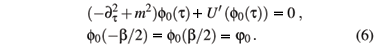We now perturb the boundary condition. We expand the classical solution fc (and then the classical action SE[fc]) as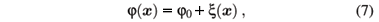and the solution of the classical equation of motion can therefore be expanded in a similar manner :where fn is of order n in x.

It is possible to show that, in order to find the classical action SE[fc] at order two in the fluctuation x(x) of the boundary (corresponding to a gaussian integral over x(x)), it is enough to obtain the classical solution fc at order one in x(x). Also, for the integration over x(x) in eq. (5) to be a Gaussian integral, we need to evaluate the determinant at lowest order in x(x), i.e. at order 0.

A. Correction to fc due to boundary fluctuations

The next step is to find the correction f1(t, x) to the classical solution fc. In our approximation, we obtain the following (linearized) equation for f1:with the boundary condition: f1(-b/2, x) = f1(b/2, x) = x(x). Using elementary calculus one can show that the first order correction to the classical solution will be, in Fourier space:with a propagator that obeys:and G(t,-b/2, k) = G(t,b/2, k) = 0. From the knowledge of two linearly independent solutions ha,b of the homogeneous linear differential equation associated to (11) it is fairly easy to determine the propagator:where W is the wronskian of {ha,hb} andThe determinant that appears in eq. (5), restricted to the space of functions that vanish at t = -b/2 and t = b/2, can be built (to order 0 in x(x)) entirely from the knowledge of the classical solution f0(t). We obtain the following non-renormalized result:B. Integration of the boundary fluctuations

The final analytic step is the calculation of the functional integral over the fluctuation x(\boldsymbol x) of the boundary. Before doing this integration, we must expand the classical action SE[fc] to quadratic order in x, using the expansion of eq. (8) for fc: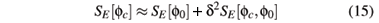where

and C(k) = ¶t ((t,t', k)) evaluated at the boundaries. Therefore, the functional gaussian integral over x(x) would lead to the following result: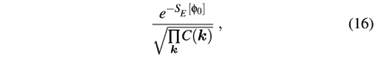that can be calculated in terms of ha and hb.

Collecting everything together, we can write the following formula for the non-renormalized partition function:where the factor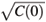arises from the fact that we do not integrate over the zero mode x(k = 0), since the uniform component goes into j0.

For the free theory the previous expression is exact. For non-trivial cases it is a non-perturbative approximation.

IV. CONCLUSION AND FINAL REMARKS

We have obtained a simple expression for the partition function of a scalar field theory in a semiclassical approximation. The ingredients have a closed form expression in terms of classical solutions for boundary conditions homogeneous in space, and it is in principle straightforward to finish the calculation of Z numerically.

In the present, we are finishing the particular case of the lf4 interaction, where we can build ha and hb analytically. We hope to reproduce hard-thermal loop results[11,12] for the pressure in the limit of high temperature.

Acknowledgments

A.B., E.S.F and C.A.A. de Carvalho acknowledge support from CAPES, CNPq, FAPERJ and FUJB/UFRJ.

 C. De-Witt-Morette, Commun. Math. Phys. 24, 47 (1972). C. De-Witt-Morette, Commun. Math. Phys. 37, 63 (1974). C. De-Witt-Morette, Ann.Phys. NY 97, 367 (1976).

 C. A. A. de Carvalho, R. M. Cavalcanti, E. S. Fraga, and S. E. Jorás, Ann. Phys. 273, 146 (1999).

 C. A. A. de Carvalho, R. M. Cavalcanti, E. S. Fraga, and S. E. Jorás, Phys. Rev. E 61, 6392 (2000).

 C. A. A. de Carvalho, Phys. Rev. D 65, 065021 (2000). C. A. A. de Carvalho, Phys. Rev. D 65, 049901 (erratum) (2000).

 A. Bessa, C. A. A. de Carvalho, and E. S. Fraga, Phys. Rev. D 69, 125013 (2004).

 A. Bessa and C. A. A. de Carvalho, J. Phys. A 39, 9891 (2006).

 F. W. Byron Jr and R. W. Fuller, Mathematics of Classical and Quantum Physics (Reading, MA:Addison-Wesley) (1970).

 R. Rajaraman, Phys. Rep. 21, 227 (1975).

 M. Le Bellac, Thermal Field Theory (Cambridge University Press) (1996)

 C. Zhai and B. Kastening, Phys. Rev. D 52, 7232 (1995).

 J. O. Andersen and M. Strickland, Ann. Phys 317, 281 (2005).

 U. Kraemmer and A. Rebhan, Rept. Prog. Phys. 67, 351 (2004).

•  C. De-Witt-Morette, Commun. Math. Phys. 24, 47 (1972).
• C. De-Witt-Morette, Commun. Math. Phys. 37, 63 (1974).
• C. De-Witt-Morette, Ann.Phys. NY 97, 367 (1976).
•  C. A. A. de Carvalho, R. M. Cavalcanti, E. S. Fraga, and S. E. Jorás, Ann. Phys. 273, 146 (1999).
•  C. A. A. de Carvalho, R. M. Cavalcanti, E. S. Fraga, and S. E. Jorás, Phys. Rev. E 61, 6392 (2000).
•  C. A. A. de Carvalho, Phys. Rev. D 65, 065021 (2000).
• C. A. A. de Carvalho, Phys. Rev. D 65, 049901 (erratum) (2000).
•  A. Bessa, C. A. A. de Carvalho, and E. S. Fraga, Phys. Rev. D 69, 125013 (2004).
•  A. Bessa and C. A. A. de Carvalho, J. Phys. A 39, 9891 (2006).
•  F. W. Byron Jr and R. W. Fuller, Mathematics of Classical and Quantum Physics (Reading, MA:Addison-Wesley) (1970).
•  R. Rajaraman, Phys. Rep. 21, 227 (1975).
•  M. Le Bellac, Thermal Field Theory (Cambridge University Press) (1996)
•  C. Zhai and B. Kastening, Phys. Rev. D 52, 7232 (1995).
•  J. O. Andersen and M. Strickland, Ann. Phys 317, 281 (2005).
•  U. Kraemmer and A. Rebhan, Rept. Prog. Phys. 67, 351 (2004).

# Publication Dates

• Publication in this collection
24 Apr 2007
• Date of issue
Mar 2007